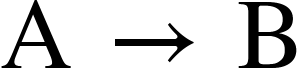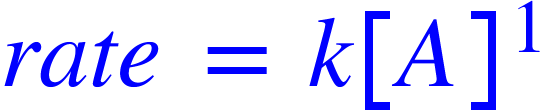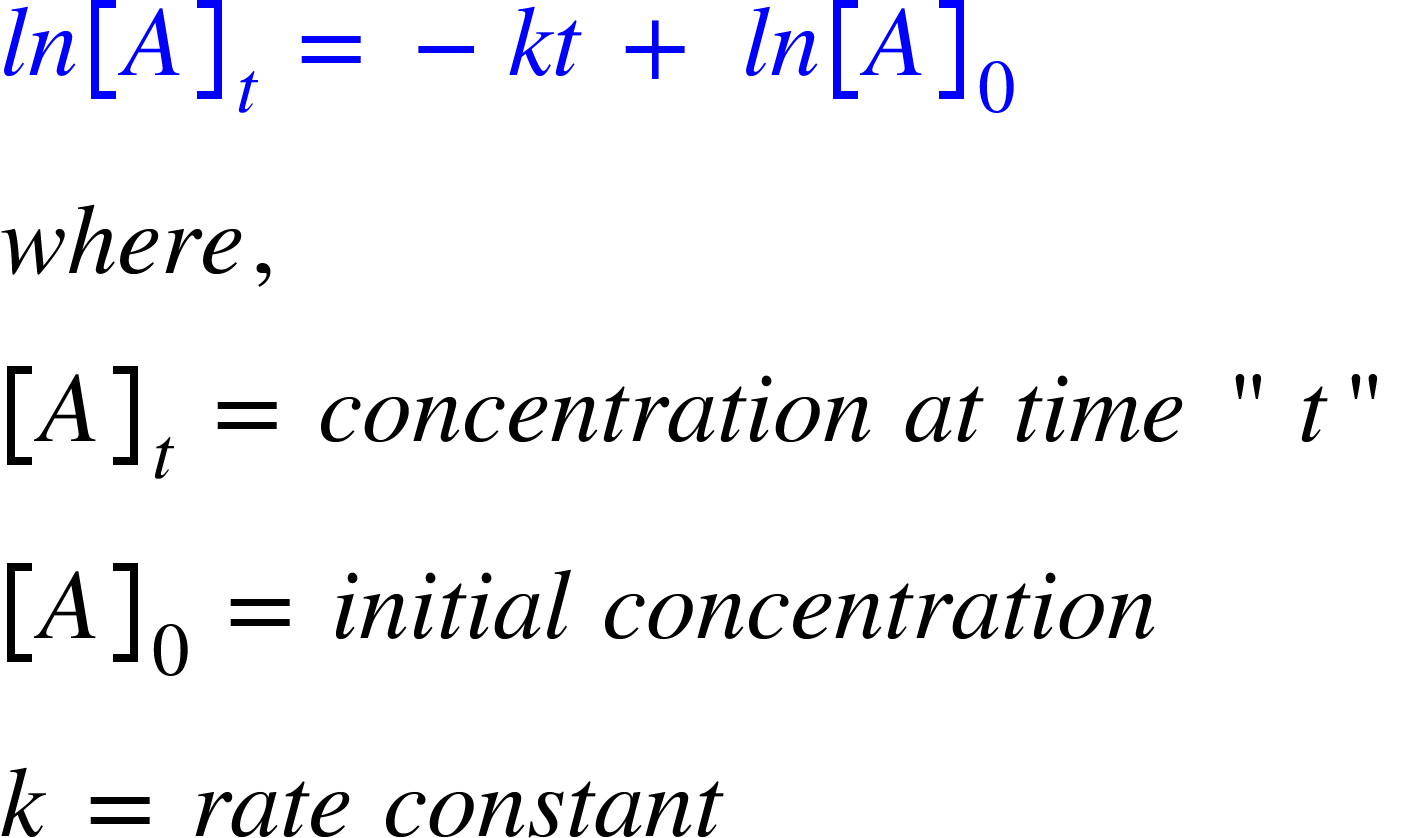# Problem: The hydrolysis of the sugar sucrose to the sugars glucose and fructose, C12H22O11 + H2O ⟶ C6H12O6 + C6H12O6 follows a first-order rate equation for the disappearance of sucrose: Rate = k[C12H22O11] (The products of the reaction, glucose and fructose, have the same molecular formulas but differ in the arrangement of the atoms in their molecules.) k = 2.1 × 10−11 s-1 at 27 °C.(b) When a solution of sucrose with an initial concentration of 0.150 M reaches equilibrium, the concentration of sucrose is 1.65 × 10−7 M. How long will it take the solution to reach equilibrium at 27 °C in the absence of a catalyst? Because the concentration of sucrose at equilibrium is so low, assume that the reaction is irreversible.

###### FREE Expert Solution

A first order reaction is a reaction whose rate depends linearly on the concentration of only one reactant. For a hypothetical reaction:Rate of reaction is given by:Integrated rate law for a first-order reaction is:We have the following data:

90% (415 ratings)###### Problem Details

The hydrolysis of the sugar sucrose to the sugars glucose and fructose, C12H22O11 + H2O ⟶ C6H12O6 + C6H12O6 follows a first-order rate equation for the disappearance of sucrose: Rate = k[C12H22O11] (The products of the reaction, glucose and fructose, have the same molecular formulas but differ in the arrangement of the atoms in their molecules.) k = 2.1 × 10−11 s-1 at 27 °C.

(b) When a solution of sucrose with an initial concentration of 0.150 M reaches equilibrium, the concentration of sucrose is 1.65 × 10−7 M. How long will it take the solution to reach equilibrium at 27 °C in the absence of a catalyst? Because the concentration of sucrose at equilibrium is so low, assume that the reaction is irreversible.